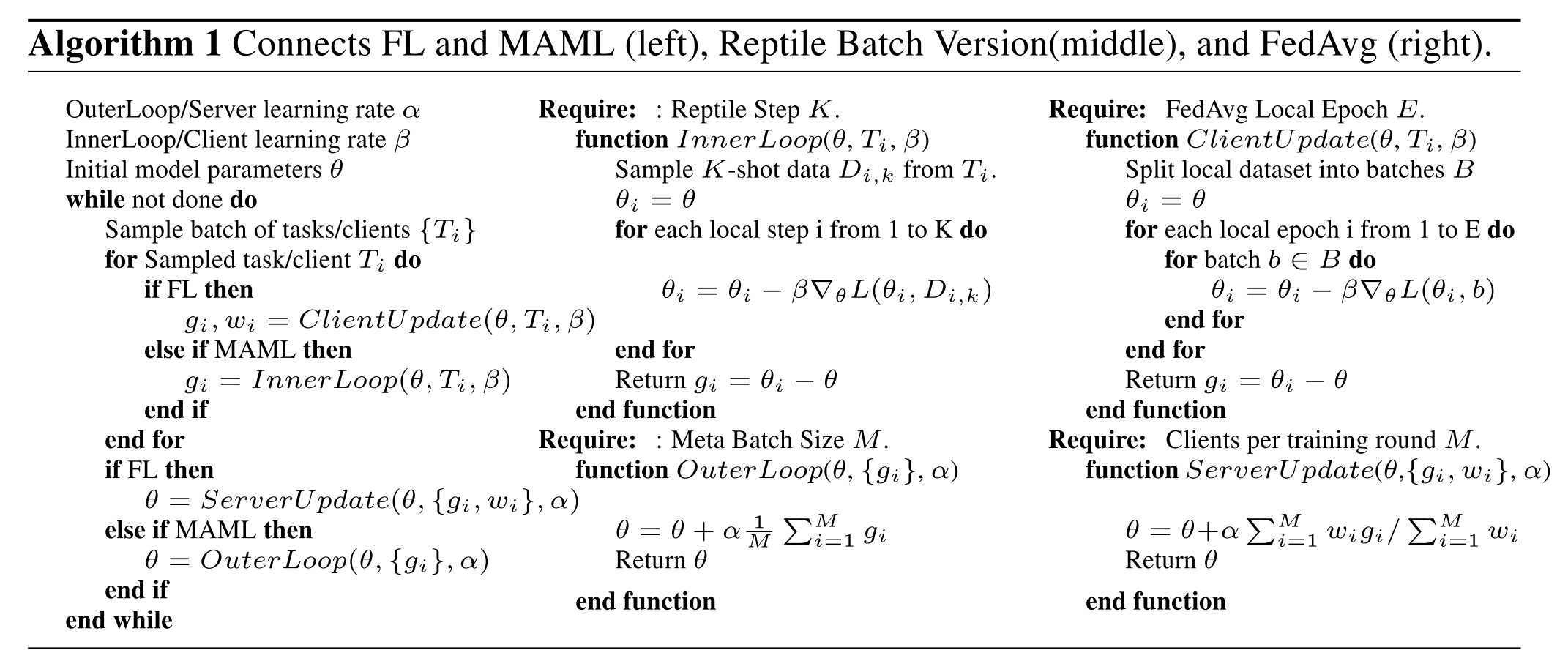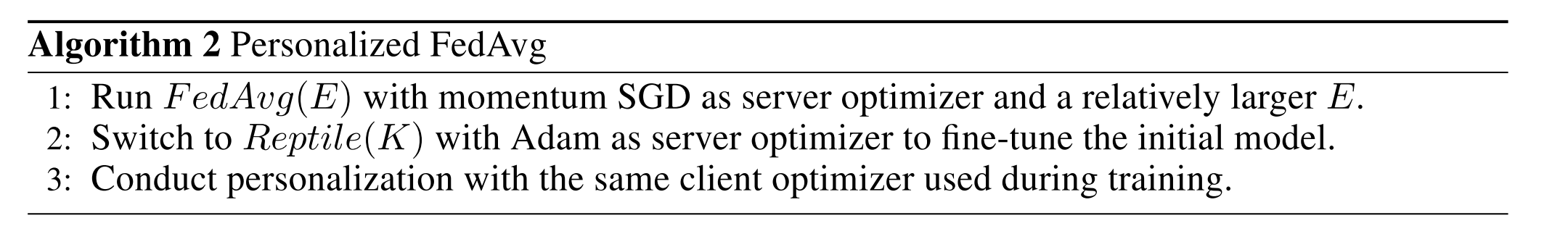• English：

# Improving Federated Learning Personalization via MAML

## Abstract

1. FL算法与MAML具有很多相似性，可以用元学习算法来对其进行解释
2. 微调可以使得gloabl 模型具有更强的准确率，同时更容易做定制化处理
3. 通过标准的中心化数据库训练出来的模型相比Fedavg训练的更难进行定制化处理

## Introduction

1. 指出了FL与MAML算法的联系，并用MAML算法对FL算法进行解释
2. 对FedAvg进行改进，采用两阶段的训练和fine-tune进行优化
3. 发现FedAvg其实本质是一种metalearning算法，用于优化个性化定制的效果，而不是全局模型的优化。

## Interpreting FedAvg as a Meta Learning AlgorithmFedSGD的梯度更新函数为：

$g_{F e d S G D}=\frac{-\beta}{T} \sum_{i=1}^{T} \frac{\partial L_{i}(\theta)}{\partial \theta}=\frac{1}{T} \sum_{i=1}^{T} g_{1}^{i}$

$\frac{\partial U_{K}^{i}(\theta)}{\partial \theta}=I-\beta \frac{\partial \sum_{j=1}^{K} g_{j}^{i}}{\partial \theta}=I-\beta \sum_{j=1}^{K} \frac{\partial^{2} L_{i}\left(\theta_{j}\right)}{\partial \theta^{2}}$

$g_{M A M L}=\frac{\partial L_{M A M L}}{\partial \theta}=\frac{1}{T} \sum_{i=1}^{T} \frac{\partial L_{i}\left(U_{K}^{i}(\theta)\right)}{\partial \theta}=\frac{1}{T} \sum_{i=1}^{T} L_{i}^{\prime}\left(U_{K}^{i}(\theta)\right)\left(I-\beta \sum_{j=1}^{K} \frac{\partial^{2} L_{i}\left(\theta_{j}\right)}{\partial \theta^{2}}\right)$

$g_{F O M A M L}(K)=\frac{1}{T} \sum_{i=1}^{T} L_{i}^{\prime}\left(U_{K}^{i}(\theta)\right) I=\frac{1}{T} \sum_{i=1}^{T} L_{i}^{\prime}\left(\theta_{K}^{i}\right)=\frac{1}{T} \sum_{i=1}^{T} g_{K+1}^{i}$

$g_{F e d A v g}=\frac{1}{T} \sum_{i=1}^{T} \sum_{j=1}^{K} g_{j}^{i}=\frac{1}{T} \sum_{i=1}^{T} g_{1}^{i}+\sum_{j=1}^{K-1} \frac{1}{T} \sum_{i=1}^{T} g_{j+1}^{i}=g_{F e d S G D}+\sum_{j=1}^{K-1} g_{F O M A M L}(j)$

## Personalized FedAvgTo be continued